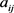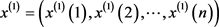# 基于因子分析和灰色预测理论的新疆综合经济实力评价 Evaluation of Xinjiang’s Comprehensive Economic Strength Based on Factor Analysis and Grey Prediction Theory

• 全文下载: PDF(485KB)    PP.649-654   DOI: 10.12677/SA.2018.76074
• 下载量: 196  浏览量: 302

Based on factor analysis method, this paper studies the comprehensive economic strength of all regions (states and cities) in Xinjiang, and selects 11 indicators reflecting the comprehensive economic strength of 15 cities (states and cities) in Xinjiang to make a comprehensive analysis. Finally, the grey prediction theory is used to forecast the comprehensive economic strength of Xinjiang from 2017 to 2021. The results show that there are differences and imbalances in the economic development of each city. Urumqi has a strong economic development advantage, ranking first in the economy, while Kirgiz Autonomous Prefecture is the weakest.

1. 理论依据

1.1. 因子分析的基本原理 

${X}_{i}={X}_{i}的共性部分+{X}_{i}独立部分\left(i=1,\cdots ,p\right)$ (1)

${X}_{i}={a}_{i1}{f}_{1}+{a}_{i2}{f}_{2}+\cdots +{a}_{im}{f}_{m}+{C}_{i}{U}_{i}$ (2)

$X=AF+CU$

(2)式中 ${a}_{i1}{f}_{1}+{a}_{i2}{f}_{2}+\cdots +{a}_{im}{f}_{m}$ 是共同元素的线性组合，它构成了 ${X}_{i}$ 共同的部分。系数反映了 ${X}_{i}$ 依赖 ${f}_{j}$ 的程度，称为 ${X}_{i}$ 在因子 ${f}_{j}$ 上的载荷，而矩阵A则称为因子载荷矩阵。

1.2. 灰色预测GM(1,1)模型的建立 

1) 记 ${x}^{\left(1\right)}$ 为生成数列：，其中， ${x}^{\left(1\right)}\left(t\right)$ 表示自原始数据的第二项开始，与本身的前一项相加。

2) 对 ${x}^{\left(1\right)}\left(t\right)$ 建立 ${x}^{\left(1\right)}\left(t\right)$ 的一阶线性微分方程： $\frac{\text{d}{x}^{\left(1\right)}}{\text{d}t}+a{x}^{\left(1\right)}=b$

3) 对于累加生成的数据需要求出其均值进而生成B和常数项向量 ${Y}_{n}$

$B=\left[\begin{array}{c}0.5\left({x}^{\left(1\right)}\left(1\right)+{x}^{\left(1\right)}\left(2\right)\right)\\ ⋮\\ 0.5\left({x}^{\left(1\right)}\left(n-1\right)+{x}^{\left(1\right)}\left(n\right)\right)\end{array}\right]$

${Y}_{n}={\left({x}^{\left(0\right)}\left(2\right),{x}^{\left(0\right)}\left(3\right),\cdots ,{x}^{\left(0\right)}\left(n\right)\right)}^{\text{T}}$

4) 通过最小二乘法，用最小误差的平方和找出数据函数的最优匹配求解灰参数 ${a}^{\prime }$${a}^{\prime }={\left({B}^{\text{T}}B\right)}^{-1}{B}^{\text{T}}{Y}_{n}$ ，将灰色参数 ${a}^{\prime }$ 代入 $\frac{\text{d}{x}^{\left(1\right)}}{\text{d}t}+a{x}^{\left(1\right)}=b$ 求解，得： ${{x}^{\prime }}^{\left(1\right)}\left(t+1\right)=\left({x}^{\left(0\right)}\left(1\right)-\frac{b}{a}\right){\text{e}}^{-at}+\frac{b}{a}$

5) 将函数 ${{x}^{\prime }}^{\left(1\right)}\left(t+1\right)$${{x}^{\prime }}^{\left(1\right)}\left(t\right)$ 进行离散，或者做差以还原 ${x}^{\left(0\right)}$ 原始序列，估计出近似数据序列：

${{x}^{\prime }}^{\left(0\right)}\left(t+1\right)={{x}^{\prime }}^{\left(1\right)}\left(t+1\right)-{{x}^{\prime }}^{\left(1\right)}\left(t\right)$

6) 利用模型进行预测： ${{x}^{\prime }}^{\left(0\right)}=\left[{{x}^{\prime }}^{\left(0\right)}\left(1\right),{{x}^{\prime }}^{\left(0\right)}\left(2\right),\cdots ,{{x}^{\prime }}^{\left(0\right)}\left(n\right),{{x}^{\prime }}^{\left(0\right)}\left(n+1\right),\cdots ,{{x}^{\prime }}^{\left(0\right)}\left(n+m\right)\right]$

2. 新疆各地州市经济发展水平的评价

2.1. 建立指标体系

2.2. 数据因子分析的结果

2.2.1. KMO检验和Bartlett检验Table 1. Inspection of KMO and bartlett

2.2.2. 计算各指标的旋转后的因子载荷矩阵，各主因子的特征值、贡献率和累计贡献率Table 2. Factor load matrix, eigenvalue, contribution rate, cumulative contribution rate after rotation

Continued

${Y}_{i}={\alpha }_{1}{F}_{i1}+{\alpha }_{2}{F}_{i2}+{\alpha }_{3}{F}_{i3}$Table 3. Ranking of comprehensive scores of cities

2.3. 基于灰色预测理论的各地(州、市)综合因子得分Table 4. Grey Prediction of Factor Score in Xinjiang from 2017 to 2021

3. 结论

1) 在第一主因子上，乌鲁木齐市的综合经济得分比其他地(州、市)要高，说明乌鲁木齐市生产总值高，居民受教育范围大，卫生医疗比较发达。这是因为乌鲁木齐市是新疆的省会，经济基础较好，经济规模和投资规模均居全区首位，为乌鲁木齐市的全面发展奠定了良好的基础。在第三主因子上，克拉玛依市得分比其他地(州、市)要高，说明克拉玛依市的工业发展比较发达，人均工资较高。由于石油资源的大规模开发带动了工业高速发展，但克拉玛依市的外来人口比例比较低，所以人均工资较高。

2) 从综合得分及排序可见，新疆各地区经济发展水平存在较大的差异，区域发展极不平衡，这一结果也基本符合新疆经济发展的实际情况。乌鲁木齐市的经济发展综合水平最高，乌鲁木齐市地域优势较好，是中国衔接中亚地区的交通枢纽，现已成为中国开展对外经济文化交流的重要窗口，所以乌鲁木齐的经济发展水平最高。而喀什地区、阿克苏地区、和田地区、塔城地区、哈密地区、吐鲁番地区、阿勒泰地区、博尔塔拉蒙古自治州、克孜勒苏柯尔克孜自治州综合得分为负值，这些地区大部分是以农业产业结构为主的城市，其经济发展水平较低，因为农业拉动经济增长的百分点持续降低，所以以农业经济为主的区域经济增长缓慢。

3) 从预测出的结果中能够看出，大部分地(州、市)的综合经济排名基本不变，在五年内的经济地位没有太大的变化，只有少数地(州、市)的综合经济排名有所变动。

  杨吉斌, 韩萍. 基于因子分析的新疆主要城市经济发展状况评价[J]. 新疆师范大学学报(自然科学版), 2009, 28(3): 64-67.  谢潇鹏. 利用灰色预测模型进行奥运会成绩预测研究[J]. 电子世界, 2018(2): 48-49.  杨维, 忠张甜, 刘荣. SPSS统计分析与行业应用案列详解[M]. 北京: 清华大学出版社, 2015: 364-369.  夏国恩, 兰政海. 基于因子分析的广西区各城市综合经济实力评价[J]. 特区经济, 2009(12): 211-213.  李辉, 李军, 刘淼. 基于主成分与灰色预测理论的新疆经济综合实力分析[J]. 兰州文理学院学报(自然科学版), 2016, 30(5): 26-31.